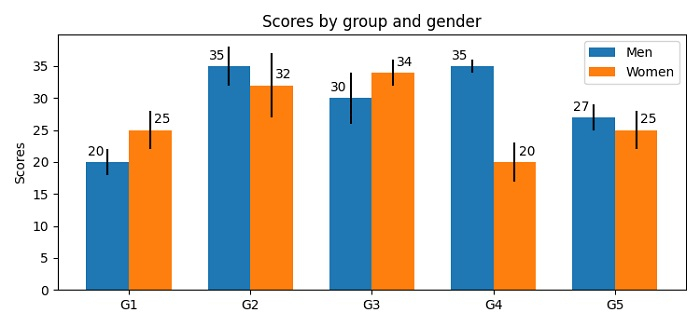# How to plot a Bar Chart with multiple labels in Matplotlib?

To plot a bar chart with multiple labels in Matplotlib, we can take the following steps −

• Make some data set for men_means, men_std, women_means, and women_std.

• Make index data points using numpy.

• Initialize the width of the bars.

• Use subplots() method to create a figure and a set of subplots.

• Create rects1 and rects2 bars rectangle using bar() method.

• Use set_ylabel(), set_title(), set_xticks() and set_xticklabels() methods.

• Place a legend on the plot.

• Add multiple labels for bar chart using autolabel() method.

• To display the figure, use show() method.

## Example

import matplotlib.pyplot as plt
import numpy as np

plt.rcParams["figure.figsize"] = [7.50, 3.50]
plt.rcParams["figure.autolayout"] = True

men_means, men_std = (20, 35, 30, 35, 27), (2, 3, 4, 1, 2)
women_means, women_std = (25, 32, 34, 20, 25), (3, 5, 2, 3, 3)

ind = np.arange(len(men_means)) # the x locations for the groups
width = 0.35 # the width of the bars

fig, ax = plt.subplots()
rects1 = ax.bar(ind - width/2, men_means, width, yerr=men_std, label='Men')
rects2 = ax.bar(ind + width/2, women_means, width, yerr=women_std, label='Women')

ax.set_ylabel('Scores')
ax.set_title('Scores by group and gender')
ax.set_xticks(ind)
ax.set_xticklabels(('G1', 'G2', 'G3', 'G4', 'G5'))
ax.legend()

def autolabel(rects, xpos='center'):
ha = {'center': 'center', 'right': 'left', 'left': 'right'}
offset = {'center': 0, 'right': 1, 'left': -1}
for rect in rects:
height = rect.get_height()
ax.annotate('{}'.format(height),
xy=(rect.get_x() + rect.get_width() / 2, height),
xytext=(offset[xpos]*3, 3), # use 3 points offset
textcoords="offset points", # in both directions
ha=ha[xpos], va='bottom')

autolabel(rects1, "left")
autolabel(rects2, "right")
plt.show()

## Output